Open In App
Related Articles
• CBSE Physics Class 8 Notes

# Contact and Non Contact Forces

Contact and Non-Contact Forces are the two main types of forces. Contact Force is a force that acts between the objects in contact with each other. For example, Contact force can be experienced when an object is sliding on a surface, due to frictional force. However, Non-Contact Force does not require any physical contact interaction. For example, when an apple falls down from a tree, because of gravitational force or pull, Some important examples of Contact Forces are Frictional Force, Air Drag (or resistance), Surface Tension, Buoyant Force, Applied Force, Normal Force, Muscular Force and so on. Similarly, some examples of Non-Contact Forces are Gravitational Force, Electrostatic Force, Magnetic Force, Nuclear force, etc.

Let’s understand the concepts of Contact and Non-Contact Force, their types and examples, the Difference between Contact and Non-Contactforces, and the FAQs on them in this article!

## What is Contact Force?

A contact force is defined as the force acting between two objects due to the physical interaction or contact between them. For example, a footballer kicks the ball is a contact force since physical contact is between the foot and the ball.

Often, contact forces are divided into two orthogonal parts: the normal force, which is perpendicular to the surface or surfaces in contact, and the friction force, which is parallel to the surface or surfaces in contact. For example, Frictional Force, Spring Force, Muscular Force, etc are contact Force.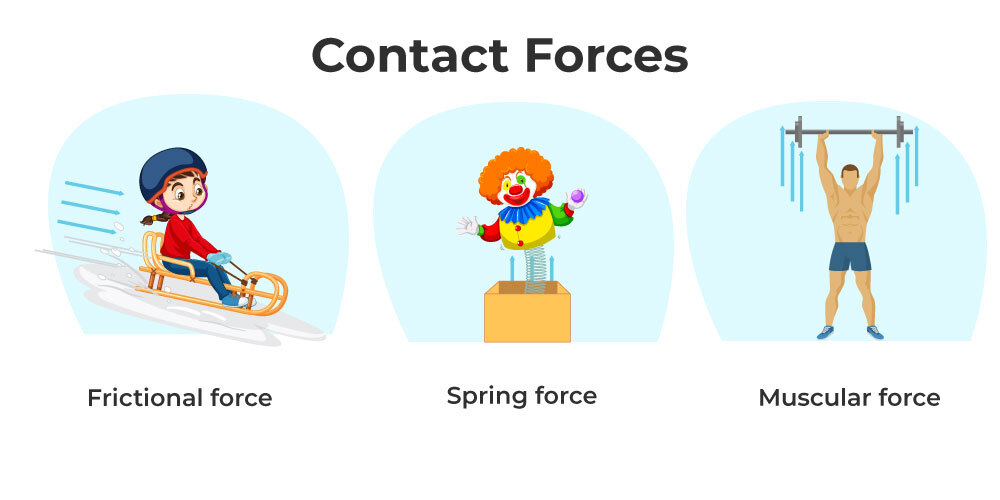## Types of Contact Forces

Contact forces are further subdivided into the following categories:

### Muscular Force

Muscles work together to create a force that is known as muscular force. Muscle force only occurs when it comes into touch with anything. We use muscular force in our daily activities such as breathing, digesting, lifting a bucket, and dragging or pushing an object. Our task is made easier by using muscular force.

### Spring Force

Spring force is the force exerted by a compressed or stretched spring. Depending on how the spring is connected, the force generated might be a push or a pull.

### Frictional Force

The frictional force operates on an item when it changes its state motion. It’s the opposing force that arises when an object is moved or attempts to move a surface. Frictional force comes from contact between two surfaces and functions as a point of contact between two surfaces. The frictional force is used to ignite a matchstick or halt a moving ball, for example. Friction has two types: sliding and static friction.

### Applied Force

When you push a table across the room, you apply a force that acts when it collides with something else. This is referred to as ‘applied force,’ which refers to a force that is applied to a person or object. There are three types of applied force: push, pull and drag force.

### Normal Force

Even if a book appears to be stationary when it is placed on a table, it is not. The book is still being acted upon by an opposing force, that of gravity, which is pushing it toward the ground. This is known as the ‘normal force.’

### Tension Force

The force exerted by a completely stretched cable or wire attached to an object is known as tension. This creates a ‘tension force,’ which pulls in both directions and applies equal pressure.

### Air Resistance

When things move through the air, they are subjected to air resistive forces, which are frictional forces. These forces are inherently resistant.

## Examples of Contact Forces

Here are the real-life examples of Contact Force, we came across in our daily life:

• A Heavy Box lying on the surface when pushed in a particular direction, then a force is created due to the interaction or contact of the box and the surface. This contact force is known as the frictional force.• When an object (say your school bag) is kept on the table, which remains at rest. The bag is in contact with the table on which applies some force on it due to its weight and gravity. Such contact force is called Applied Force.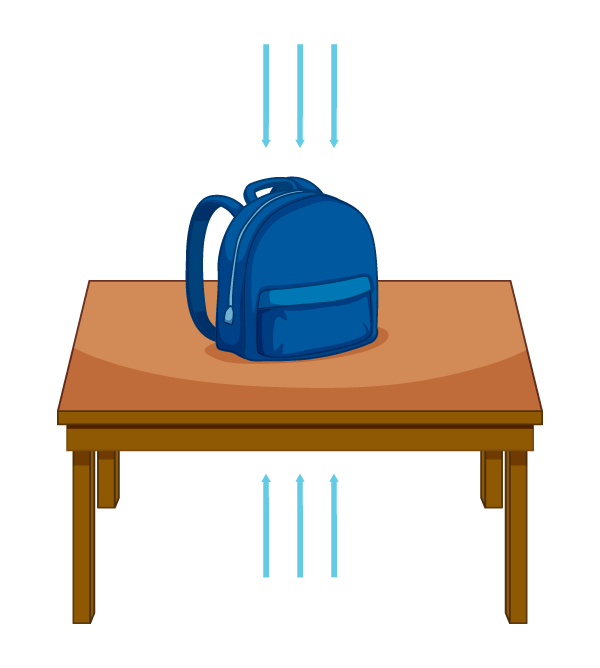• When an airplane is flying, it moves forward in the air due to its contact with the air. Such contact force is called Air Drag or resistance.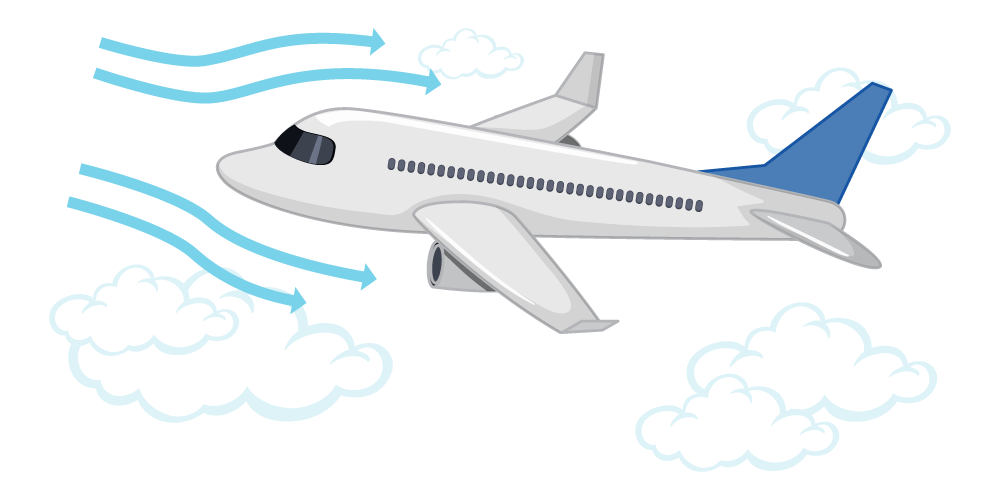## Non-Contact Forces

Non-contact forces are the forces that acts on the object, without any physical interaction with them, For example, when a ball is thrown upward came back to the thrower due to gravity due to its weight.

There are a lesser number of non-contact forces in comparison to contact forces. Some examples of non-contact forces are gravitational force, electrostatic force, Magnetic force, and Nuclear forces.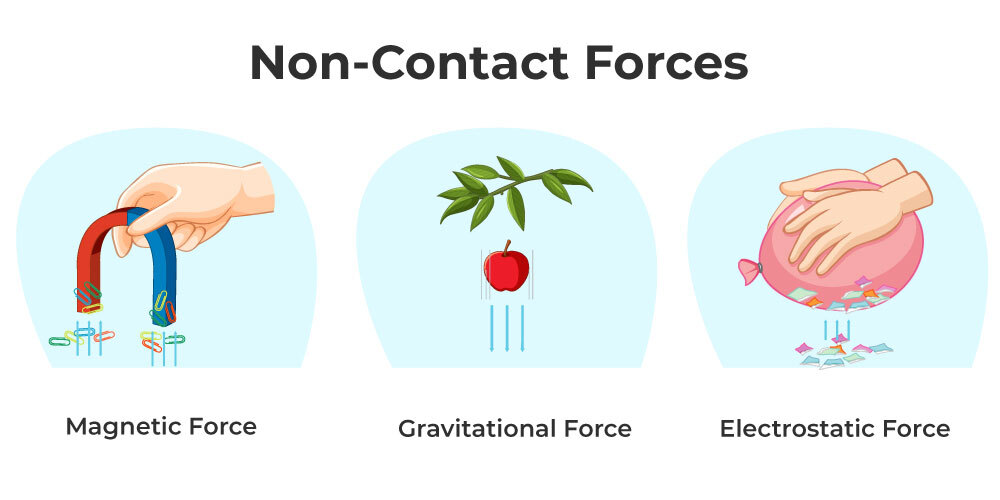## Types of Non-Contact Forces

They are further subdivided into the following forces:

### Gravitational Force

Newton’s law of gravity says that “gravitational forces between two bodies are directly equal to the product of their masses and inversely proportional to the square of the distance between them.” Large things, such as planets and stars, exert this force.

### Electrostatic Force

Electrostatic forces are the sorts of forces that all electrically charged bodies in the cosmos exert on other electrically charged substances. Based on the charge of the bodies, these forces can be both attractive and repulsive in nature.

### Magnetic Force

Magnetic forces are the sorts of forces exerted by a magnet on magnetic objects. They exist in the absence of any physical interaction between two things.

## Examples of Non-Contact Forces

Let’s now understand more about the Non-Contact Forces, through the following examples:

• When a comb is rubbed on Hairs, it gains some static charging, so attracts paper pieces. This is due to a non-contact force called Electrostatic Force.• A parachutist falling freely in the air, experiences an attraction or pull toward the center of the earth due to the Non-contact force known as Gravitational Force.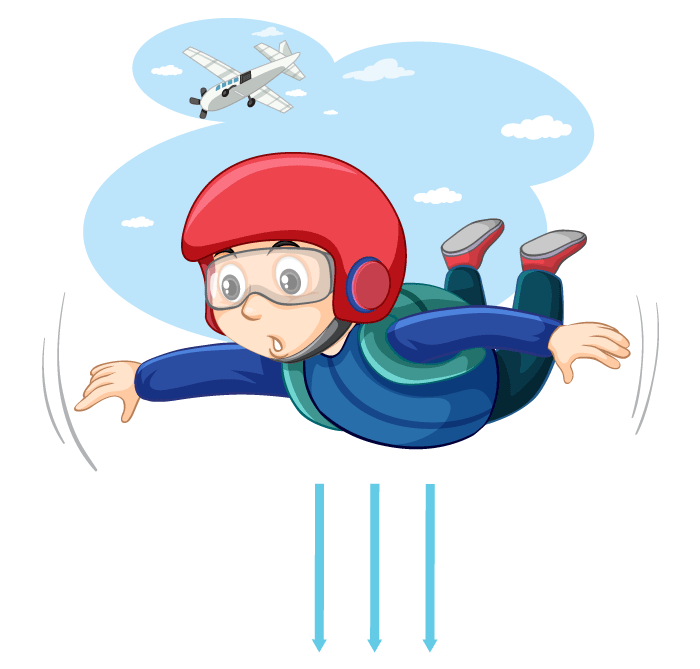## FAQs on Contact and Non-Contact Forces

Question 1: What are Contact and non-contact forces? Explain with Examples.

A contact force is defined as the force acting between two objects due to the physical interaction or contact between them. For example, a footballer kicks the ball is a contact force since physical contact is between the foot and the ball.

Non-contact forces are the forces that acts on the object, without any physical interaction with them, For example, when a ball is thrown upward came back to the thrower due to gravity due to its weight.

Question 2: Write 4 differences between contact and non-contact forces.

The following are the difference between contact and non-contact forces:

Question 3: Define Normal Force.

Normal force is a contact force. If two surfaces are not in contact, they can’t exert a normal force on each other.

Question 4: How does Inertia Prevent Motion from Changing?

Solution:

We known that every object has a special characteristics called inertia. Therefore, if you roll a ball in a vacuum (a place free of gravity and external force), it keeps rolling. If the medium is changed to air, the ball will now fall to the earth due to an external force, specifically air resistance and gravity.

Question 5: Falling raindrops an example of Contact or Non-Contact Force?

Solution:

Falling raindrops towards the earth is due to gravitational force, which is a Non-Contact Force.